## Example Questions

### Example Question #1 : How To Find The Length Of A Side Of A Polygon

If the following shape was going to be drawn in a circle, what is the minimum radius of the circle?

9

7

8

10

11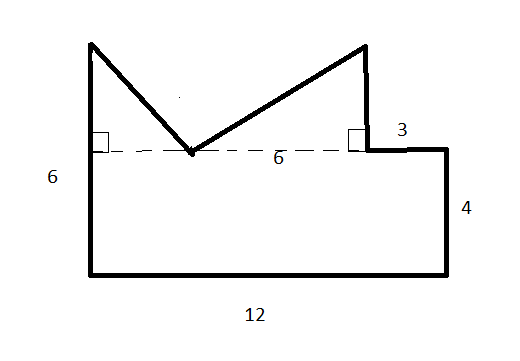7

Explanation:

IF you draw the longest diagonal across the shape, the length of it is 13.4. This means the radius must be at least 6.7. The answer is 7.

### Example Question #2 : How To Find The Length Of A Side Of A Polygon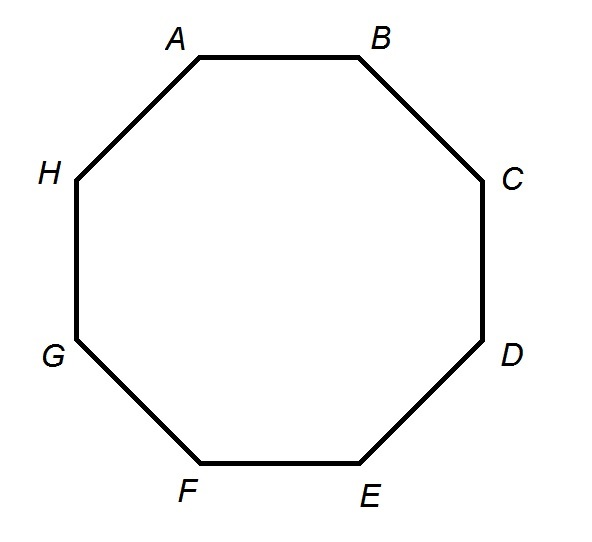Each side of the above octagonal track is 264 feet in length. Julie starts at point A and runs clockwise at a steady speed of nine miles an hour for nine minutes. When she is finished, which of the following points is closest to her?

Point D

Point C

Point G

Point E

Point F

Point D

Explanation:

Julie runs for nine minutes, or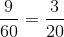hour; she runs nine miles per hour. Setting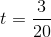and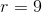in the rate formula, we can evaluate distance in miles: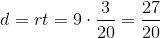Julie runs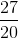miles, which converts to feet by multiplication by 5,280 feet per mile: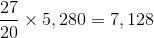feet.

Each side of the octagonal track measures 264 feet, so Julie runs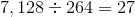sides of the track; this is equivalent to running the entire track three times, then three more sides. She is running clockwise, so three more sides from Point A puts her at Point D. This is the correct response.

### Example Question #3 : How To Find The Length Of A Side Of A Polygon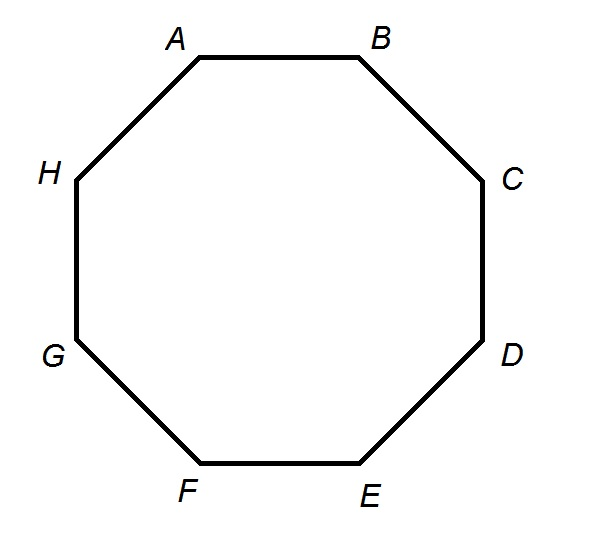Plato High School has an unusual track in that it is shaped like a regular octagon. The track has a perimeter of two-fifths of a mile.

Boris starts at Point A and runs clockwise until he gets halfway between Point E and Point F. Which of the following responses comes closest to the number of feet he runs?

1,400 feet

1,500 feet

1,200 feet

1,100 feet

1,300 feet

1,200 feet

Explanation:

One mile comprises 5,280 feet; the perimeter of the track, two-fifths of a mile, is equal to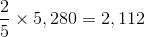feet.

Each (congruent) side of the octagonal track measures one-eighth of this,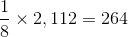feet.

By running clockwise from Point A to halfway between Point E and Point F, Boris runs along four and one half sides, each of which has this length, for a total running distance of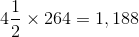feet.

Of the five responses, 1,200 comes closest.